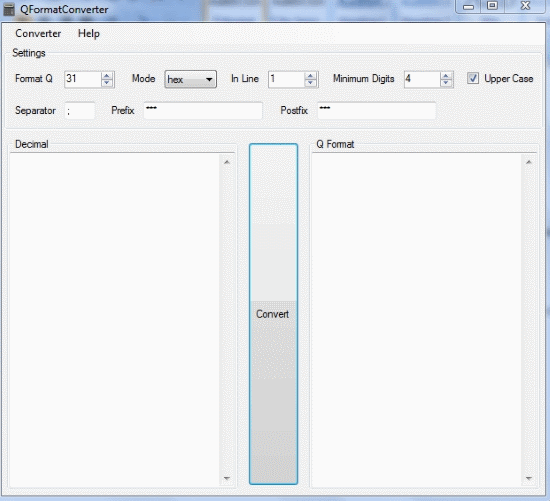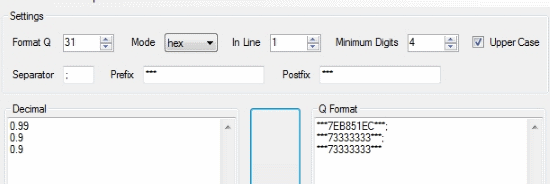Editor Ratings:
User Ratings:
[Total: 5 Average: 5]

QFormatConverter is a free format configurable calculator that can convert a list of decimal values into a Q fixed point number format. The Q format numbers are used in Digital Signal Processing calculations. These are fixed point numbers that are stored and operate like regular signed binary integers.  These help standard integer hardware and logical units to perform rational number calculations.

QFormatConverter is one of its kind that can convert decimal numbers with variable fraction bits (upto 31). I honestly couldn’t find any software online that can convert decimal numbers into a Q format notation. Not only that, you can change the result in a decimal base as well as in a hexadecimal base. You can also change the number of digits in the hexadecimal conversion.QformatConverter lets you add postfix and prefix text too, just in case you want to personalize the result to fit with wherever you are posting the result. If you already have a file with a list of decimal values that you want to convert, you can load the file and covert it in Q format with QFormatConverter.

So let’s see how QFormatConverter works. You might also want to try out scientific calculators for your DSP calculations too. You can check them out here.

## Convert a Decimal into Q format using QFormatConverter

To convert a decimal number into Q format notation, you have to follow the following steps:

1. Enter the decimal number in the space provided
2. Next, you have to choose the number of fractional bits by entering it into Format Q.
3. Select the mode of answer, choosing Hex will answer the Q format in hexadecimal. Dec is for the decimal notation
4. Choose the number of converted results per line by inputting it in the In Line field.
5. Choose the minimum number of digits of hexadecimal result by entering the same in Minimum Digits
6. You can add predefined text too. The Separator will separate the results with whatever text you enter in it. Filling the Prefix and Postfix fields will fix the entered text before and after the result respectivelyClick on convert in the middle of the interface to start the conversion. You will get the results on the left in the Q Format text area.

## Load a File to Convert using QFormatConverter

You can also convert a big text file with decimals in a list that have to be converted. On the menu, click Conveter>Load and select the desired file to be converted. Make sure the decimals that need to be converted are in a column list in the file. You can also save the result, click on Save to save it.

## Final Words

The software does what it is intended to do. Now, you don’t have to manually calculate the Q format of a decimal number. The fact that you can load a long list of decimal values to convert makes it an excellent choice. I give this software 5 stars.

Get QFormatConverter here.

Know a numeral base converter that includes Q format too? Do tell me in the comments!